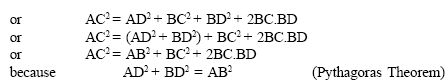Name: ___________________Date:___________________

kwizNET Subscribers, please login to turn off the Ads!
 Email us to get an instant 20% discount on highly effective K-12 Math & English kwizNET Programs!

### High School Mathematics - 28.14 Pythagorean Theorem - Examples

 Theorem: In a right angled triangle, the square on the hypotenuse is equal to the sum of the squares of the other two sides. If the length of the hypotnuse is c and the lengths of other two sides are b and a then according to the pythogorean theorem: c2 = a2 + b2Converse: In a triangle if the square of one side is equal to the squares on the sums of the other two sides, then the angle opposite to the first side is a right angle. To prove: AB2 + BC2 = AC2Example: Prove that the sum of the squares of the sides of a rhombus is equal to the sum of the squares of the diagonals.Directions: Solve the following problems. Also write at least 10 examples of your own.
 Q 1: A person goes 10 m due east and then 30m due north. Find his distance from the starting point.15m10m12m Q 2: Which of these is a Pythagorean triplet?13, 12, 58, 9, 1315, 8, 5 Q 3: Two poles 6m and 11m stand on a plane ground. If the distance between their feet is 12m, determine the distance between their tops.12 m11 m13 m Q 4: A ladder is placed in such a way such that its distance from the wall to the foot of the ladder is 5 cm, its top reaches a height of 12m from the ground. Find the length of the ladder.15m12m13m Q 5: A ladder 20 m long reaches a height of 16m above the ground. Find the distance from the foot of the ladder to the wall.13 m17 m12 m Q 6: The sides of a triangle are 7cm, 24cm, 25cm, check if this is a right angled triangle.noyes Question 7: This question is available to subscribers only! Question 8: This question is available to subscribers only!

#### Subscription to kwizNET Learning System costs less than \$1 per month & offers the following benefits:

• Unrestricted access to grade appropriate lessons, quizzes, & printable worksheets
• Instant scoring of online quizzes
• Progress tracking and award certificates to keep your student motivated
• Unlimited practice with auto-generated 'WIZ MATH' quizzes
• Child-friendly website with no advertisements

© 2003-2007 kwizNET Learning System LLC. All rights reserved. This material may not be reproduced, displayed, modified or distributed without the express prior written permission of the copyright holder. For permission, contact info@kwizNET.com
For unlimited printable worksheets & more, go to http://www.kwizNET.com.